## Introduction to Statistical Thinking

2019-7-1

Ph.D.:       University of California, Los Angeles

Master:     University of California, Los Angeles

Bachelor:  Beijing Institute of Technology.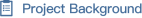A person’s orthopedic condition can be detected  from his/her biomechanical features. Advances in medical devices made possible biomechanical measurements for patients who bear potential orthopedic abnormalities. Data mining techniques can be utilized to perform disease detection and phenotyping when applied towards biomechanical data. The project is based on a dataset originated from the vertebral column dataset in UCI machine learning repository1, built by Dr. Henrique da Mota during a medical residency in the Group of Applied Research in Orthopedics (GARO) in France. Six numerical biomechanical features are derived from the shape and orientation of the pelvis and lumbar spine: pelvic incidence, pelvic tilt, lumbar lordosis angle, sacral slope, pelvic radius, grade of spondylolisthesis. Accurately classifying orthopedic conditions using easily acquired measurements will contribute to prompt diagnosis and effective targeted treatments.

The instructor has 3 years’ teaching assistant experience in statistics, R/Python programming and mathematical modeling at UCLA for lower-class undergraduate courses and have led multiple data analytics final projects. This course will equip the student with basic knowledge and coding techniques to perform quantitative analysis on a research problem with results presented as deliverables upon completion.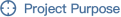1.Introduction to basic concepts in statistics and data mining methods

2.Introduction to coding in R

3.The student is expected to perform exploratory analysis on a real-life data set, apply appropriate data mining algorithms to perform classification task and interpret the results.

4. The student will be exposed to English scientific paper reading, summary, and scientific report writing.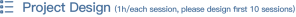The following sessions are designed for the fundamental knowledge of the project. Student has to understand all the details of it before moving on to the next stage

 Time Schedule Content 0h-2h Introduction to statistics and data mining: basic concepts and motivating examplesLab: R installation, main interface, package installation, documentation, variable assignment, naming convention, math calculation, basic data types in R, data I/O, variable typesProject: How to ask research questions for a given data set? Read example scientific papers. pick a domain and a dataset. 2h-4h Probability theory: ● what is probability● how do we determine probabilities?● probability distributions● conditional probability● computing cond. probability● Independence● Odds and odds ratioPractice: calculate probabilities and conditional probabilitiesProject: Background, literature search 4h-6h Understanding the data: what is data, exploratory analysis, summary statistics, understand the equations and calculate by hand, distributionsLab: table indexing/subsetting/combination, summary statistics, data visualization (plots), for loopProject: Perform exploratory analysis on the chosen dataset, what conclusions can you draw from the data? Read example scientific papers. 6h-8h Data preprocessing: normalization, imputation, removing abnormal values.Lab: normalization, imputation, removing abnormal values, writing a functionProject: preprocessing the given dataset 8h-10h Modeling categorical relationships: Example study, Pearson’s Chi-squared test,  contingency tables and the two-way test, odds ratios, Simpson’s ParadoxProject: how can this be applied to your dataset? 10h-12h Modeling continuous relationships: Example study, covariance and correlation, correlation and causationProject: how can this be applied to your dataset? 12h-14h Generalized linear models: linear regression, multivariate linear regression, interactions between variables, cross-validationLab: fitting a linear model, perform cross-validation 14h-16h (Comparing means: hypothesis testing )Reproducible researchScientific report writing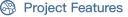a. It will introduce the student to basic concepts in statistics and probability theory

b. The data analysis routine introduced and the coding techniques are transferable to future data analysis projects.

c. Cultivate scientific statistical thinking and literature reading skills.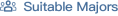Data mining, Informatics, Statistics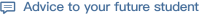This course is designed for pre-college level students. The student is expected to perform literature summary, accomplish assigned tasks to reinforce statistical concepts and draft up the final report offline, under guidance when appropriate. Critical thinking will be promoted throughout the course, while the student is encouraged to investigate ‘why’ and ‘what if’ independently. Curiosity is the best teacher.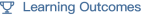At the end of the course, the student will:

1. Be familiar with the data analytics routine

2. Know basic concepts of statistics and probability, able to perform calculation by hand/in R.

3. Build confidence in performing basic coding in R.

4. Explore application background and current techniques in the chosen domain